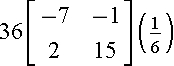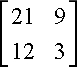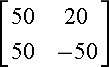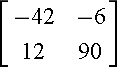Multiplying Matrices by Scalar

Matrix Multiplication

By Scalar

The product of a real number x, called a scalar, and a matrix M is a new matrix xM, where xM stands for the multiplication of all the elements of matrix M by scalar x.

Example

Multiply the scalar 5 by the matrix .

Solution

Properties of Scalar Multiplication

Let M and N be xy matrices, and let m and n be scalars. The following are the properties of scalar multiplication:
1. Associative Property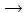(mn)(M) = m(nM)
2. Distributive Property(+ n)M = mM + nM
3. Distributive Propertym(MN) = mM + mN
4. Scalar Identity1M = M
5. Associative Propertym(M)n = mn(M)

Exercises

1.)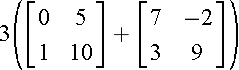2.)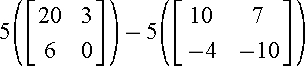3.)